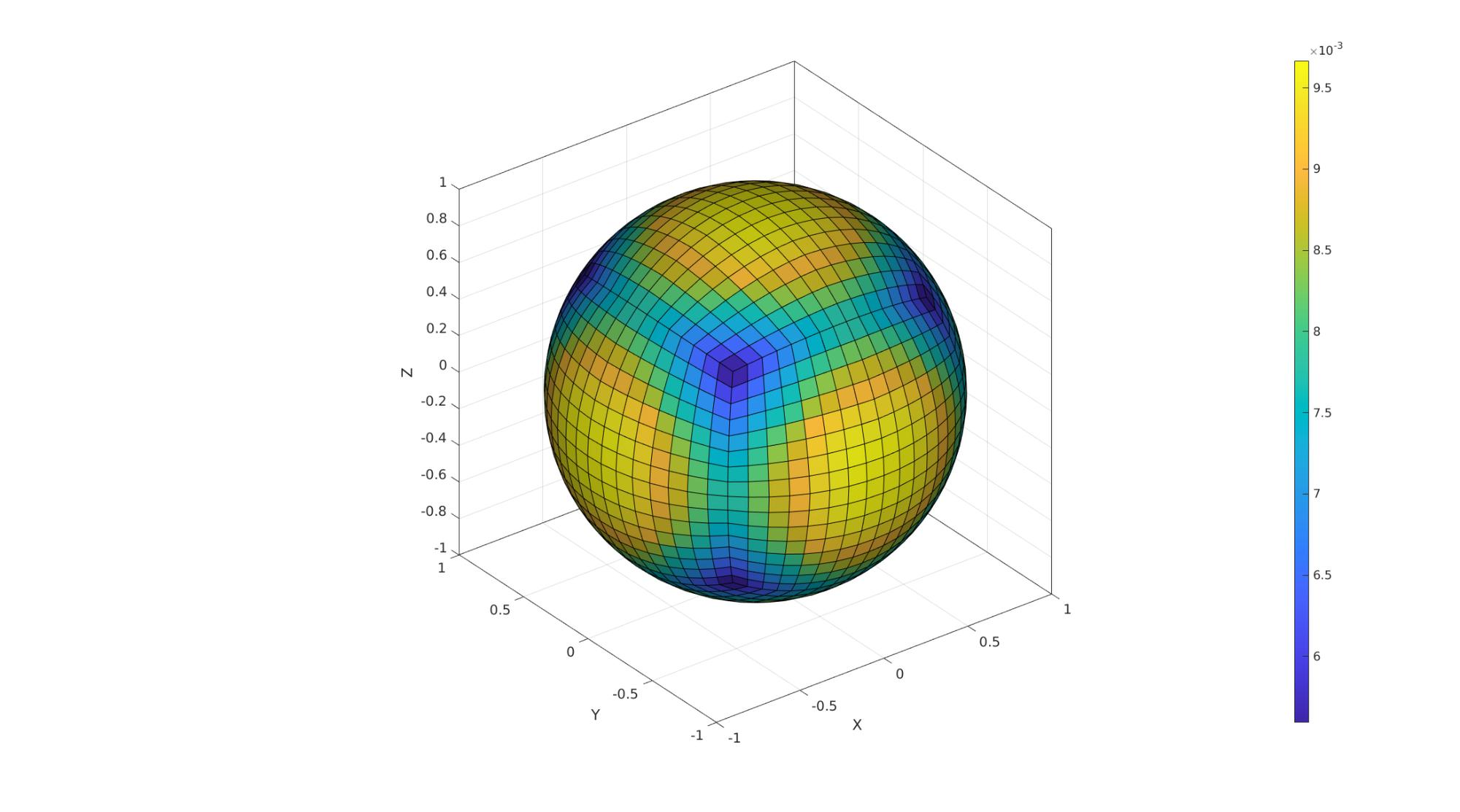# patchArea

Below is a demonstration of the features of the patchArea function

## Contents

```clear; close all; clc;
```

## Syntax

[A]=patchArea(F,V);

## Description

The patchArea function computes the surface area of the patch elements defined by the faces F and vertices V.

## Examples

```testCase=5;
switch testCase
case 1 %Circle
r=1;
n=250;
t=linspace(-pi,pi,n)'; t=t(1:end-1);
V=[r*cos(t) r*sin(t) zeros(size(t))];
F=[1:size(V,1)];
aTotalTrue=pi*r^2 %True theoretical area
case 2 %Single element square 1x1
V=[0 0 0; 1 0 0; 0 1 0; 1 1 0];
F=[1 2 4 3];
aTotalTrue=1 %True theoretical area
case 3 %Multi element square rotated 1x1
V1=0.5*[-1 -1; -1 1; 1 1; 1 -1];
regionCell={V1}; %A region between V1 and V2 (V2 forms a hole inside V1)
plotOn=0; %This turns on/off plotting
pointSpacing=0.1; %Desired point spacing
resampleCurveOpt=1; %Option to turn on/off resampling of input boundary curves
[F,V]=regionTriMesh2D(regionCell,pointSpacing,resampleCurveOpt,plotOn);
V(:,3)=0;
aTotalTrue=1 %True theoretical area
R=euler2DCM([-0.25*pi 0.25*pi 0]);
V=V*R;
case 4 %Cube
[V,F]=platonic_solid(2);
V=V./max(V(:))/2;
aTotalTrue=6 %True theoretical area
r=1;
n=4;
% [F,V]=geoSphere(n,r);
aTotalTrue=4*pi*r^2 %True theoretical area
case 6 %Sphere triangles
r=1;
n=4;
[F,V]=geoSphere(n,r);
aTotalTrue=4*pi*r^2 %True theoretical area
end
```
```aTotalTrue =

12.5664

```
```%Compute areas for each patch/face
[A]=patchArea(F,V);

%Checking sum with theoretical (not changes due to discrete nature are
%possible).
aTotalEstimate=sum(A(:)) %Summed area check
```
```aTotalEstimate =

12.5400

```

Visualization

```cFigure;
gpatch(F,V,A);
colormap parula; colorbar;
gdrawnow;
```GIBBON www.gibboncode.org

Kevin Mattheus Moerman, [email protected]

GIBBON footer text

GIBBON: The Geometry and Image-based Bioengineering add-On. A toolbox for image segmentation, image-based modeling, meshing, and finite element analysis.

Copyright (C) 2006-2021 Kevin Mattheus Moerman and the GIBBON contributors

This program is free software: you can redistribute it and/or modify it under the terms of the GNU General Public License as published by the Free Software Foundation, either version 3 of the License, or (at your option) any later version.

This program is distributed in the hope that it will be useful, but WITHOUT ANY WARRANTY; without even the implied warranty of MERCHANTABILITY or FITNESS FOR A PARTICULAR PURPOSE. See the GNU General Public License for more details.

You should have received a copy of the GNU General Public License along with this program. If not, see http://www.gnu.org/licenses/.